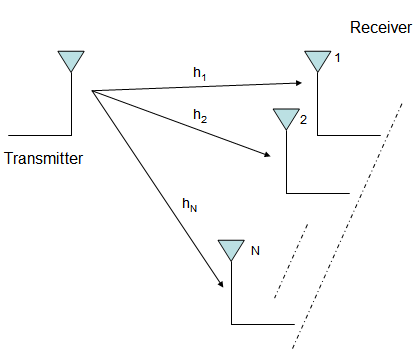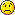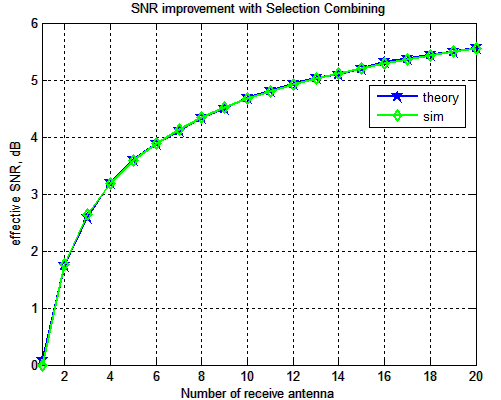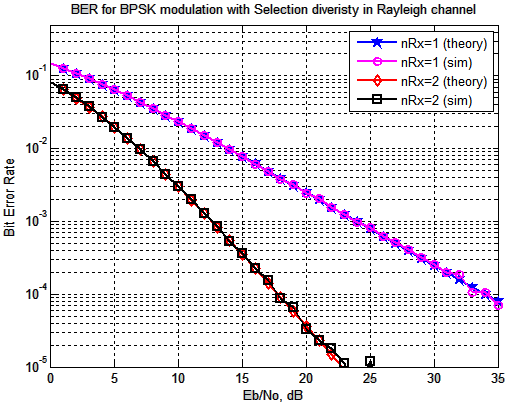- DSP log - http://www.dsplog.com -

Selection Diversity

Posted By Krishna Sankar On September 6, 2008 @ 2:44 pm In MIMO | 60 Comments

This is the first post in the series discussing receiver diversity in a wireless link. Receiver diversity is a form of space diversity, where there are multiple antennas at the receiver. The presence of receiver diversity poses an interesting problem – how do we use ‘effectively‘ the information from all the antennas to demodulate the data. There are multiple ways to approach the problem. The three typical approaches to be discussed are – selection diversity, equal gain combining and maximal ratio combining. In this post we will discuss selection diversity. For the discussion, we will assume that the channel is a flat fading Rayleigh multipath channel  and the modulation is BPSK.

## Background

1. We have N receive antennas and one transmit antenna.

2. The channel is flat fading – In simple terms, it means that the multipath channel has only one tap. So, the convolution operation reduces to a simple multiplication. For a more rigorous discussion on flat fading and frequency selective fading, may I urge you to review Chapter 15.3 Signal Time-Spreading from [DIGITAL COMMUNICATIONS: SKLAR]3. The channel experienced by each receive antenna is randomly varying in time. For the receive antenna, each transmitted symbol gets multiplied by a randomly varying complex number . As the channel under consideration is a Rayleigh channel, the real and imaginary parts of are Gaussian distributed having mean 0 and variance 1/2.

4. The channel experience by each receive antenna is independent from the channel experienced by other receive antennas.

5. On each receive antenna, the noise has the Gaussian probability density function with

with and .

The noise on each receive antenna is independent from the noise on the other receive antennas.

6. At each receive antenna, the channel is known at the receiver. For example, on the receive antenna, equalization is performed at the receiver by dividing the received symbol by the apriori known i.e.

where
is the additive noise scaled by the channel coefficient.

7. In the presence of channel , the instantaneous bit energy to noise ratio at receive antenna is . For notational convenience, let us define,

.

From the discussion on chi-square random variable , we know that, if is a Rayleigh distributed random variable, then is a chi-squared random variable with two degrees of freedom. The pdf of is
.

## What is selection diversity?

Consider a scenario where we have a single antenna for transmission and multiple antennas at the receiver (as shown in the figure below).At the receiver we have now N copies of the same transmitted symbol. Which then poses the problem – how to effectively combine them to reliably recover the data.

Selection diversity approach is one way out – With selection diversity, the receiver selects the antenna with the highest received signal power and ignore observations from the other antennas. The chosen receive antenna is one which gives .

## Outage probability in Selection Diversity

The equations in the post refers the note on Receive diversity  by Prof. RaviRaj Adve.

To analyze the bit error rate, let us first find the outage probability on the receive antenna. Outage probability is the probability that the bit energy to noise ratio falls below a threshold. The probability of outage on receive antenna is,

.

is the defined threshold for bit energy to noise ratio.

In N reveive antenna case, the probability that all bit energy to noise ratio on all the receive antenna are below the threshold is,

,

where

are the bit energy to noise ratio on the 1st, 2nd and so on till the Nth receive antenna.

Since the channel on each antenna is assumed to independent, the joint probability is the product of individual probabilities.

.

Note that the equation above defines the probability that the effective bit energy to noise ratio with N receive antennas (lets call ) is lower than the threshold . This is infact the cumulative distribuition function (CDF) of . The probability density function (PDF) is then the deriviate of the CDF..

Given that we know the PDF of , the average output bit energy to noise ratio is,

.

I do not know how to reduce the above integral to this simple sum.This means that,

- with two receive antennas the effective bit energy to noise ratio is 1.5 times ,

- with three receive antennas, the effective bit energy to noise ratio is 1.833 times ,

- with four receive antennas, the effective bit energy to noise ratio is 2 times and so on.

If you recall the results from the AWGN with receive diversity case ,

Effective bit energy to noise ratio in a N receive antenna case is N times the bit energy to noise ratio for single antenna case.

With selection diversity we are seeing that the effective SNR improvement is not a linear improvement with increasing the number of receive antennas. The returns diminish.Figure: SNR gain with selection diversity

## Bit Error probability with selection diversity

If you recall, in the post on BER computation in AWGN , with bit energy to noise ratio of , the bit error rate for BPSK in AWGN is derived as

.

Given that the effective bit energy to noise ratio with selection diversity is, the total bit error rate is the integral of the conditional BER integrated over all possible values of .

.

This equation reduces to

.

[DIG-COMM-BARRY-LEE-MESSERSCHMITT].  Again, I do not know the proof## BER Simulation Model

The Matlab/Octave script performs the following

(a) Generate random binary sequence of +1′s and -1′s.

(b) Multiply the symbols with the channel and then add white Gaussian noise.

(d) Chose that receive path, equalize (divide) the received symbols with the known channel

(d) Perform hard decision decoding and count the bit errors

(e) Repeat for multiple values of and plot the simulation and theoretical results.Figure: BER plot for BPSK in Rayleigh channel with Selection Diversity

## Observations

Around 16dB improvement at BER point by with two receive antenna selection diversity## References

[DIG-COMM-BARRY-LEE-MESSERSCHMITT] Digital Communication: Third Edition, by John R. Barry, Edward A. Lee, David G. MesserschmittHappy learning.

URLs in this post:

 Rayleigh multipath channel: http://www.dsplog.com/2008/07/14/rayleigh-multipath-channel/

 chi-square random variable: http://www.dsplog.com/2008/07/28/chi-square-random-variable/#twodegree